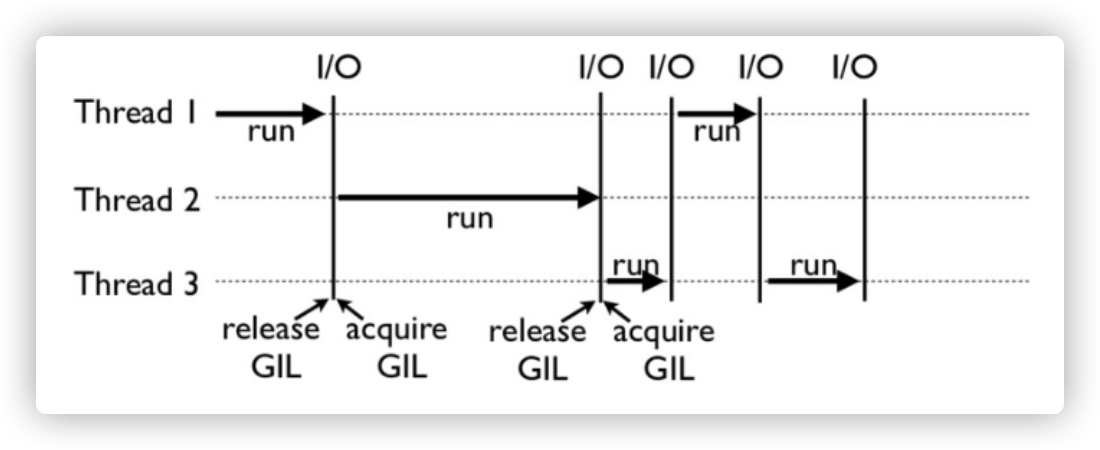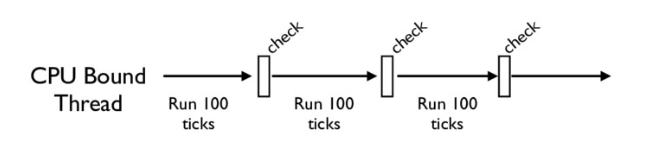## 1. 引言

### 1.1 为什么变慢了？

import time
def Countnumber(n):
while n > 0:
n -= 1
start = time.time()
Countnumber(100000000)
end = time.time()
print('运行时间为：{}秒'.format(end-start))
# 输出



import time
N = 100000000
def Countnumber(n):
while n > 0:
n -= 1

start = time.time()
t1.start()
t2.start()
t3.start()
t4.start()
t1.join()
t2.join()
t3.join()
t4.join()
end = time.time()
print('运行时间为：{}秒'.format(end-start))
# 输出



## 2. GIL

GIL是Python自带解释器，也是最流行的Python解释器CPython中的一个技术，它的中文名为：全局解释器锁，每个Python线程，在CPython解释器中执行的时候，都会先锁住自己的线程，阻止别的线程执行。

Python的垃圾回收机制是以引用计数为主，标记-清除和分代回收为辅的策略。

import sys
a = []
b = a
print(sys.getrefcount(a))
# 输出
3


• 为了规避内存管理的race condition(竞争)问题
• 顾名思义，CPython就是使用C解释Python语言，而大部分C语言库都不是原生线程安全的

## 3. GIl是如何工作的？CPython中的check_interval机制会轮训检查线程GIL的锁情况，每隔一段时间，Python解释器就会强制当前的线程去释放GIL，这样别的线程才能有机会去执行。

Python3中，CPython会在一个“合理”的范围内释放GIL（以Python3为例，interval的时间大概是15毫秒）for (;;) {
if (--ticker < 0) {
ticker = check_interval;

/* Give another thread a chance */

/* Other threads may run now */

}

bytecode = *next_instr++;
switch (bytecode) {
/* execute the next instruction ... */
}
}


## 4. Python的线程安全

import threading

n = 0
def foo():
global n
n += 1

for i in range(1000):
t.start()
t.join()
print(n)

import dis
print(dis.dis(foo))
# 输出
6 STORE_GLOBAL             0 (n)
10 RETURN_VALUE
None


n = 0
def foo():
global n
with lock:
n += 1


## 5. 如何绕过GIL？

• 绕过CPython，使用IPython或者JPython（Java实现的Python解释器）等解释器实现；
• 把对于性能要求高的代码，放到别的语言中实现；

# 6.奇怪的想法

import time
import multiprocessing
N = 100000000
def Countnumber(n):
while n > 0:
n -= 1

start = time.time()
t1 = multiprocessing.Process(target=Countnumber,args=[N // 2])
t2 = multiprocessing.Process(target=Countnumber,args=[N // 2])

t1.start()
t2.start()

t1.join()
t2.join()

end = time.time()
print('运行时间为：{}秒'.format(end-start))
# 输出



Q.E.D.

print("种一棵树最好的时间是十年前，其次是现在")